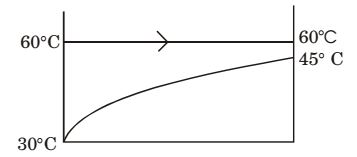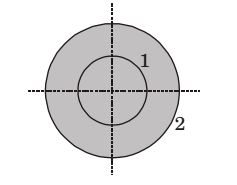## Heat Transfer Miscellaneous

#### Heat-Transfer

1. A spherical steel ball of 12 mm diameter is initially at 1000 K. It is slowly cooled in a surrounding of 300 K. The heat transfer coefficient between the steel ball and the surroundings is 5 W/m²K. The thermal conductivity of steel is 20 W/mK. The ternperature difference between the centre and the surface of the steel ball is

1.  Bi = hL = 5 ×0.002 = 0.0005 K 20

For the given condition, Biot number tends to zero, i.e. conduction resistance is far less than convection resistance. Therefore temperature between centre and surface is very small.

##### Correct Option: D

 Bi = hL = 5 ×0.002 = 0.0005 K 20

For the given condition, Biot number tends to zero, i.e. conduction resistance is far less than convection resistance. Therefore temperature between centre and surface is very small.

1. Water (c = 4.18 kJ/kgK) at 80°C enters a counter flow heat exchanger with a mass flow rate of 0.5 kg/s. Air (c = 1 kJ/kgK) enter at 30°C with a mass flow rate 2.09 kg/s. If the effectiveness of the heat exchanger is 0.8, the LMTD (in °C) is

1.  ε = Ch × (th1 - th2) Cmin (th1 - tc1)

 ∴ 0.8 = 4.18 × 0.5 × (80 - th2) 2.09 × 1 (80 - 30)

⇒ th2 = 40°C
mhcph (th1 – th2) = mc ccp (tc2 – tc1)
∴ 0.5 × 4.18 × 40 = 2.09 × 1 × (tc2 – 30)
⇒ tc2 = 70°C
&thet;1 = θ2 = 10°C
∴ LMTD = 10°C

##### Correct Option: C

 ε = Ch × (th1 - th2) Cmin (th1 - tc1)

 ∴ 0.8 = 4.18 × 0.5 × (80 - th2) 2.09 × 1 (80 - 30)

⇒ th2 = 40°C
mhcph (th1 – th2) = mc ccp (tc2 – tc1)
∴ 0.5 × 4.18 × 40 = 2.09 × 1 × (tc2 – 30)
⇒ tc2 = 70°C
&thet;1 = θ2 = 10°C
∴ LMTD = 10°C

1. In a condenser of a power plant, the steam condenses at a temperature of 60°C. The cooling water enters at 30°C and leaves at 45°C. The Logarithmic Mean Temperature Difference (LMTD) of the condenser is

1. Flow configuration in condenser as shown below.ΔT1 = 30°C (Q1)
ΔT2 = 15°C (Q2)

 LMTD = ΔT1 - ΔT2 = 30 - 15 InΔT1In30ΔT2 15

= 21.6°C

##### Correct Option: B

Flow configuration in condenser as shown below.ΔT1 = 30°C (Q1)
ΔT2 = 15°C (Q2)

 LMTD = ΔT1 - ΔT2 = 30 - 15 InΔT1In30ΔT2 15

= 21.6°C

1. For the same inlet and exit temperatures of the hot and cold fluids, the log mean temperature difference (LMTD) is

1. NA

##### Correct Option: B

NA

1. Consider two infinitely long thin concentric tubes of circular cross section as shown in figure. If D1 and D2 are the diameters of the inner and outer tubes respectively, then the view factor F22 is given by1. F22 = 1 - F21 =

 = 1 - A1 = 1 - D1 A2 D2

##### Correct Option: D

F22 = 1 - F21 =

 = 1 - A1 = 1 - D1 A2 D2